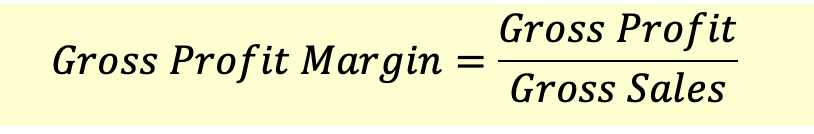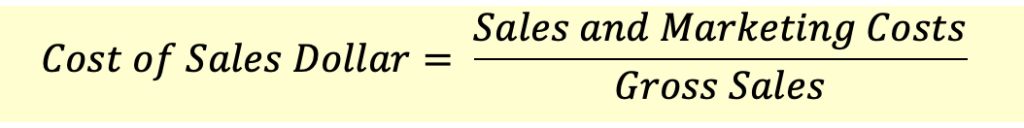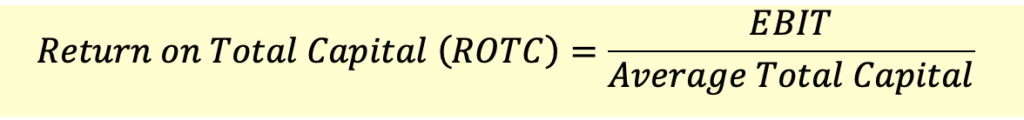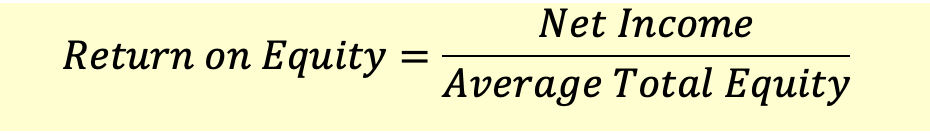# Profitability Ratios

Profitability ratios measure how profitable a company is.

### Gross Profit MarginGross profit margin measures gross profit as a percentage of gross revenue. This helps capture profit as a percentage of revenue, and helps assess components that affect profitability.

### Net Profit Margin

When net profit or the bottom line, profit after subtracting all costs is shown as a percentage of gross sales we call it net profit margin.This margin varies from industry to industry. For example a 5-6% profit in the steel manufacturing industry is considered very good, but poor for the pharmaceutical industry. It also needs to be compared with the firm’s historical performance.

### Cost per Sales Dollar

This helps estimate sales and marketing as a percentage of Gross Sales. This helps in cost control.We will now look at the profitability ratios that look at profits relative to the funds invested in the business (Return on Investment).

### Return on Assets

This measure calculates the returns as a percentage of total assets. It uses net income as the numerator. This ratio tells us how much returns the company generated from the invested capital.One problem with this measure is that the denominator (total assets) contains both debt and equity. However, the numerator (net income) excludes interest. For this reason, sometimes the denominator is adjusted by adding back the interest expense net of taxes.### Operating Return on Assets (ROA)

Operating ROA is calculated just like Return on Assets but uses Earnings Before Interest and Taxes (EBIT) instead on Net Income.Operating return on assets indicates the company's operating income generated per dollar invested in total assets. A higher operating RoA is preferred and while analysing this ratio, the analyst must analyse the historical performance and also compare it with the peers in the industry.

### Return on Total Capital

This ratio is calculated by dividing the EBIT by the total capital that includes long-term debt, short-term debt, preferred equity and common equity.This is an important profitability measure. By including debt in the total capital, the analyst is able to assess how well the management of the company is utilizing the funds available to them. A higher ROTC is preferred and should be atleast above the firm’s average borrowing rate.

### Return on Equity (ROE)

This ratio is calculated by dividing the Net Income by the Average Total Equity. The ratio tells us how much returns shareholder’s earned on their investment.This is one of the most widely used ratios by investors. However, since this ratio does not include debt, looking at just this ratio may be misleading. A company with very high debt will have a small equity base and even a small amount of net income will produce high ROE. Therefore, this ratio must be analysed together with other profitability measures.

This ratio is also sometimes called Return on Total Equity.

### Return on Common Equity

This is a variation of ROE, and calculates the returns earned only by the common shareholders. We consider only the profits available to common shareholders and equity invested by common shareholders instead of all shareholders.Get our Data Science for Finance Bundle for just $29$51.